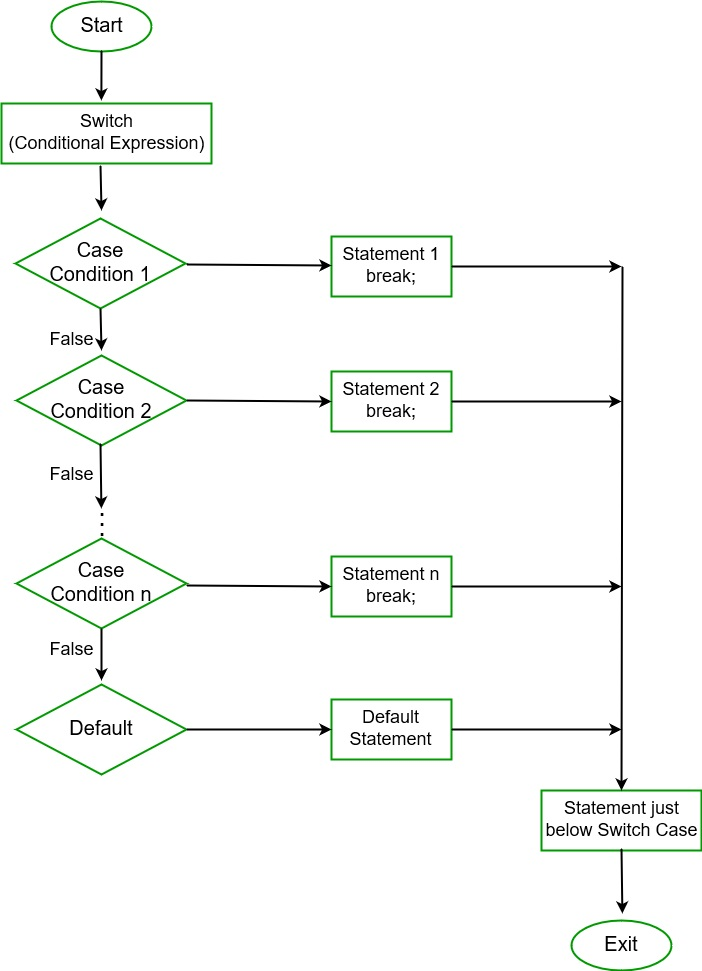# Switch Statement in C#

• Difficulty Level : Easy
• Last Updated : 22 Apr, 2020

In C#, Switch statement is a multiway branch statement. It provides an efficient way to transfer the execution to different parts of a code based on the value of the expression. The switch expression is of integer type such as int, char, byte, or short, or of an enumeration type, or of string type. The expression is checked for different cases and the one match is executed.

Syntax:

```switch (expression) {

case value1: // statement sequence
break;

case value2: // statement sequence
break;
.
.
.
case valueN: // statement sequence
break;

default:    // default statement sequence
}
```

Flow Chart:Important points to remember:

• In C#, duplicate case values are not allowed.
• The data type of the variable in the switch and value of a case must be of the same type.
• The value of a case must be a constant or a literal. Variables are not allowed.
• The break in switch statement is used to terminate the current sequence.
• The default statement is optional and it can be used anywhere inside the switch statement.
• Multiple default statements are not allowed.

Example:

 `// C# program to illustrate``// switch case statement``using` `System;`` ` `public` `class` `GFG {`` ` `    ``// Main Method``    ``public` `static` `void` `Main(String[] args)``    ``{``        ``int` `nitem = 5;``        ``switch` `(nitem) {`` ` `        ``case` `1:``            ``Console.WriteLine(``"case 1"``);``            ``break``;`` ` `        ``case` `5:``            ``Console.WriteLine(``"case 5"``);``            ``break``;`` ` `        ``case` `9:``            ``Console.WriteLine(``"case 9"``);``            ``break``;`` ` `        ``default``:``            ``Console.WriteLine(``"No match found"``);``            ``break``;``        ``}``    ``}``}`
Output:
```case 5
```

#### Why do we use Switch Statements instead of if-else statements?

We use a switch statement instead of if-else statements because if-else statement only works for a small number of logical evaluations of a value. If you use if-else statement for a larger number of possible conditions then, it takes more time to write and also become difficult to read.

Example: Using if-else-if statement

 `// C# program to illustrate``// if-else statement``using` `System;`` ` `class` `GFG {`` ` `    ``// Main Method``    ``public` `static` `void` `Main(String[] args)``    ``{``        ``// taking two strings value``        ``string` `topic;``        ``string` `category;`` ` `        ``// taking topic name``        ``topic = ``"Inheritance"``;`` ` `                ``// using compare function of string class``        ``if` `((String.Compare(topic, ``"Introduction to C#"``) == 0) || ``            ``(String.Compare(topic, ``"Variables"``) == 0) || ``            ``(String.Compare(topic, ``"Data Types"``) == 0))``        ``{``            ``category = ``"Basic"``;``        ``}``         ` `                ``// using compare function of string class``        ``else` `if` `((String.Compare(topic, ``"Loops"``) == 0) || ``                 ``(String.Compare(topic, ``"If Statements"``) == 0) || ``                 ``(String.Compare(topic, ``"Jump Statements"``) == 0)) ``        ``{``            ``category = ``"Control Flow"``;``        ``}``             ` `                ``// using compare function of string class``        ``else` `if` `((String.Compare(topic, ``"Class & Object"``) == 0) || ``                 ``(String.Compare(topic, ``"Inheritance"``) == 0) || ``                 ``(String.Compare(topic, ``"Constructors"``) == 0)) ``        ``{``            ``category = ``"OOPS Concept"``;``        ``}``         ` `        ``else` `        ``{``            ``category = ``"Not Mentioned"``;``        ``}`` ` `        ``System.Console.Write(``"Category is "` `+ category);``    ``}``}`
Output:
```Category is OOPS Concept
```

Explanation: As shown in the above program the code is not excessive but, it looks complicated to read and took more time to write. So we use a switch statement to save time and write optimized code. Using switch statement will provide a better readability of code.

Example: Using Switch Statement

 `// C# program to illustrate``// switch statement``using` `System;`` ` `class` `GFG {`` ` `    ``// Main Method``    ``public` `static` `void` `Main(String[] args)``    ``{``        ``// taking two strings value``        ``string` `topic;``        ``string` `category;`` ` `        ``// taking topic name``        ``topic = ``"Inheritance"``;``         ` `        ``// using switch Statement``        ``switch``(topic)``        ``{``            ``case` `"Introduction to C#"``:``            ``case` `"Variables"``:``            ``case`  `"Data Types"``:``                  ` `                ``category = ``"Basic"``;``                ``break``;``                 ` `             ``case` `"Loops"``:``             ``case``"If Statements"``:``             ``case``"Jump Statements"``:``                  ` `                ``category = ``"Control Flow"``;``                ``break``;``                 ` `             ``case` `"Class & Object"``:``             ``case` `"Inheritance"``:``             ``case` `"Constructors"``:``                  ` `                ``category = ``"OOPS Concept"``;``                ``break``;``                 ` `             ``// default case ``             ``default``:``                ``category = ``"Not Mentioned"``;``                ``break``;``                 ` `        ``}``         ` `        ``System.Console.Write(``"Category is "` `+ category);``    ``}``}`
Output:
```Category is OOPS Concept
```

#### Using goto in the Switch Statement

You can also use goto statement in place of the break in the switch statement. Generally, we use a break statement to exit from the switch statement. But in some situations, the default statement is required to be executed, so we use the goto statement. It allows executing default condition in the switch statement. The goto statement is also used to jump to a labeled location in C# program.

Example:

 `// C# program to illustrate the``// use of goto in switch statement``using` `System;`` ` `public` `class` `GFG {`` ` `// Main Method``public` `static` `void` `Main(String[] args)``    ``{``        ``int` `greeting = 2;`` ` `        ``switch` `(greeting) {``        ``case` `1:``            ``Console.WriteLine(``"Hello"``);``            ``goto` `default``;``        ``case` `2:``            ``Console.WriteLine(``"Bonjour"``);``            ``goto` `case` `3;``        ``case` `3:``            ``Console.WriteLine(``"Namaste"``);``            ``goto` `default``;``        ``default``:``            ``Console.WriteLine(``"Entered value is: "` `+ greeting);``            ``break``;``        ``}``    ``}``}`
Output:
```Bonjour
Namaste
Entered value is: 2
```

Explanation: In the above program, the goto statement is used in a switch statement. Here first the case 2, i.e Bonjour is printed because the switch contains the value of greeting is 2, then the control transfers to the case 3 due to the presence of goto statement, so it prints Namaste and in last the control transfer to the default condition and print Entered value is: 2.

Note: You can also use continue in place of a break in switch statement if your switch statement is a part of a loop, then continue statement will cause execution to return instantly to the starting of the loop.

My Personal Notes arrow_drop_up